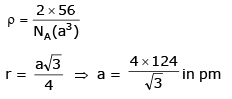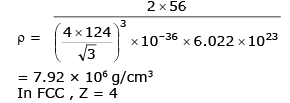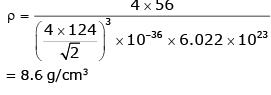JEE  >  Solid State MCQ - 1 (Advanced)

# Solid State MCQ - 1 (Advanced)

Test Description

## 25 Questions MCQ Test Chemistry for JEE Advanced | Solid State MCQ - 1 (Advanced)

Solid State MCQ - 1 (Advanced) for JEE 2022 is part of Chemistry for JEE Advanced preparation. The Solid State MCQ - 1 (Advanced) questions and answers have been prepared according to the JEE exam syllabus.The Solid State MCQ - 1 (Advanced) MCQs are made for JEE 2022 Exam. Find important definitions, questions, notes, meanings, examples, exercises, MCQs and online tests for Solid State MCQ - 1 (Advanced) below.
 1 Crore+ students have signed up on EduRev. Have you?
*Multiple options can be correct
Solid State MCQ - 1 (Advanced) - Question 1

### Select the correct statement (s)

Detailed Solution for Solid State MCQ - 1 (Advanced) - Question 1

(A) 6 Cs+ ion second nearest neighbour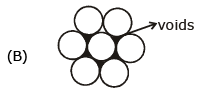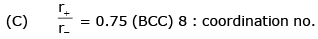(D) True

*Multiple options can be correct
Solid State MCQ - 1 (Advanced) - Question 2

### The compound having general formula M2+ Fe2O42_ are called as :

Detailed Solution for Solid State MCQ - 1 (Advanced) - Question 2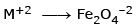it will be ferrite and ferrimagnetic substance

*Multiple options can be correct
Solid State MCQ - 1 (Advanced) - Question 3

### Which of the following statement is/are incorrect in the rock-salt structure if an ionic compounds ?

Detailed Solution for Solid State MCQ - 1 (Advanced) - Question 3

Coordination no. of cation = 6
coordination no of anion = 6

*Multiple options can be correct
Solid State MCQ - 1 (Advanced) - Question 4

Which of the following statements are correct :

Detailed Solution for Solid State MCQ - 1 (Advanced) - Question 4

r+ + r = a/2
(95 + 181)= a/2
⇒ a = 276 × 2
a = 552 pm

*Multiple options can be correct
Solid State MCQ - 1 (Advanced) - Question 5

Passage

In two dimension, there are square close packing & Hexagonal close packing. In three dimension, however threre are HCP, BCC and CCP arrangement. Unoccupied space in packing is known as void which may be octahedral, tetrahedral or cubic void and formation of void depends on atomic or ionic arrangment in crystal.

Q.

Empty space left in HCP in three dimension is

Detailed Solution for Solid State MCQ - 1 (Advanced) - Question 5

H C P
Pacling efficlency = 74%
empty space = 100 – 74%
ES=26%

*Multiple options can be correct
Solid State MCQ - 1 (Advanced) - Question 6

In two dimension, there are square close packing & Hexagonal close packing. In three dimension, however threre are HCP, BCC and CCP arrangement. Unoccupied space in packing is known as void which may be octahedral, tetrahedral or cubic void and formation of void depends on atomic or ionic arrangment in crystal.

Q.

In ccp, ratio of no. of atoms to no. of tetrahedral void is -

Detailed Solution for Solid State MCQ - 1 (Advanced) - Question 6

In any structure if number of atom is Z
so no. of tetrahedral voids = 2 Z
in ccp Z = 4
so letrahedral void = 8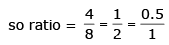*Multiple options can be correct
Solid State MCQ - 1 (Advanced) - Question 7

In two dimension, there are square close packing & Hexagonal close packing. In three dimension, however threre are HCP, BCC and CCP arrangement. Unoccupied space in packing is known as void which may be octahedral, tetrahedral or cubic void and formation of void depends on atomic or ionic arrangment in crystal.

Q.

Size order of octahedral, tetrahedral & cubic void is -

Detailed Solution for Solid State MCQ - 1 (Advanced) - Question 7

For B.C.C.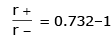for octahedral.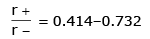for tetrahedral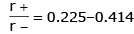so, cubic > octahedral > tetrahedral

*Multiple options can be correct
Solid State MCQ - 1 (Advanced) - Question 8

In two dimension, there are square close packing & Hexagonal close packing. In three dimension, however threre are HCP, BCC and CCP arrangement. Unoccupied space in packing is known as void which may be octahedral, tetrahedral or cubic void and formation of void depends on atomic or ionic arrangment in crystal.

Q.

In certain metallic oxide, metal M occupy HCP lattice & O_2 occupy 2/3rd of octahedral void, formula of crystal is -

Detailed Solution for Solid State MCQ - 1 (Advanced) - Question 8

M— H.C.P = 6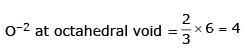M6O4
M3O2

*Multiple options can be correct
Solid State MCQ - 1 (Advanced) - Question 9

Statement - I. Due to Frenkel defect the density of solid decrease.

Statement - II. In Frenkel defect cation or anion is present at interstitial site leaving lattice sites vacant.

Detailed Solution for Solid State MCQ - 1 (Advanced) - Question 9

A Frenkel defect is a type of defect in crystalline solids wherein an atom is displaced from its lattice position to an interstitial site, creating a vacancy at the original site and an interstitial defect at the new location within the same element without any changes in chemical properties.

No cation are anion are leaving the crystal. Only the cation is moving from one place to another place within the crystal. So the density of crystalline solid will not change.

*Multiple options can be correct
Solid State MCQ - 1 (Advanced) - Question 10

Statement - I. By increasing the temperature C.N. of CsCl changes from 8 : 8 to 6 : 6.

Statement - II. Temperature also influences the C.N. of solid.

Detailed Solution for Solid State MCQ - 1 (Advanced) - Question 10

CSCl has b.c.c
by increasing temperature it will become octahedral type and it C.N. will be 6
Staterment - I is correct and statement II is correct explanation of I So answer will be (A)

*Multiple options can be correct
Solid State MCQ - 1 (Advanced) - Question 11

Statement - I. Solid having more F-centers have intense color.

Statement - II. Excess of Na+ in NaCl solid having F-centers make it appears to pink.

Detailed Solution for Solid State MCQ - 1 (Advanced) - Question 11

statment I is correct
because F- certres are the electron occupied at the place of anion which is released by metal thus it will give intense colour whereas statement II is wrong

*Multiple options can be correct
Solid State MCQ - 1 (Advanced) - Question 12

Statement - I. Close packing of atoms in cubic structure is in order of : fcc > bcc > sc

Statement - II. Packing density =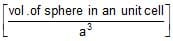Detailed Solution for Solid State MCQ - 1 (Advanced) - Question 12

F.C.C. = 74%
B.C.C. = 68%
SC= 52%
So FCC > BCC > SC
So statement I is correct
Statement II correct.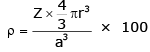and correct explanation of I

*Multiple options can be correct
Solid State MCQ - 1 (Advanced) - Question 13

Statement - I. Pure NaCl is diamagnetic in nature.

Statement - II. In NaCl, Na+ occupy octahedral voids.

Detailed Solution for Solid State MCQ - 1 (Advanced) - Question 13

Statement I - No unpaired electron in NaCl so diamagnetic
Statemetn II - is correct but not correct exblanation of I so

Solid State MCQ - 1 (Advanced) - Question 14

State whether the following statements are True or False :

Coluor of LiCl due to metal excess defect is pink.

Detailed Solution for Solid State MCQ - 1 (Advanced) - Question 14

In case we caused oping lithium goes in interstitiel site whcih cause pink colour True.

Solid State MCQ - 1 (Advanced) - Question 15

State whether the following statements are True or False :

The imperfection in crystal increases with increases in temperature.

Detailed Solution for Solid State MCQ - 1 (Advanced) - Question 15

As we increase the temperature effect will also increase.

Solid State MCQ - 1 (Advanced) - Question 16

State whether the following statements are True or False :

FCC structure possesses the most close packing of atoms in it.

Detailed Solution for Solid State MCQ - 1 (Advanced) - Question 16

FCC and ccp has the highest packing efficiency

Solid State MCQ - 1 (Advanced) - Question 17

State whether the following statements are True or False :

Increase in pressure in ionic solids may decrease co-ordination No.

Detailed Solution for Solid State MCQ - 1 (Advanced) - Question 17

Increase in pressure increase coordination number

Solid State MCQ - 1 (Advanced) - Question 18

State whether the following statements are True or False :

The amorphous solids are isotropic.

Detailed Solution for Solid State MCQ - 1 (Advanced) - Question 18

The amporsphous solid are isotropic.

Solid State MCQ - 1 (Advanced) - Question 19

Match the type of packing given in Column-I with the items given in Column-II.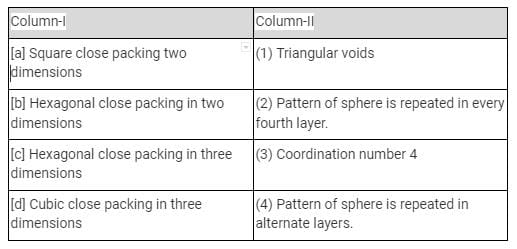Detailed Solution for Solid State MCQ - 1 (Advanced) - Question 19

Coordination number is 4 in square close packing in two dimensions. Two dimensional hexagonal close packing contains triangular voids. Three dimensional hexagonal close packing have ABAB arrangement whereas ccp have ABCAABC type arrangement.

Solid State MCQ - 1 (Advanced) - Question 20

A cubic solid is made up of two elements A and B. Atoms B are at the corners of the cube and A at the body centre. What is the formula of compound.

Detailed Solution for Solid State MCQ - 1 (Advanced) - Question 20

A = 1 (Body center)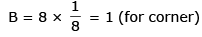formula = AB

Solid State MCQ - 1 (Advanced) - Question 21

A compound alloy of gold and copper crystallizes in a cubic lattice in which gold occupy that lattice point at corners of the cube and copper atom occupy the centres of each of the cube faces. What is the formula of this compound.

Detailed Solution for Solid State MCQ - 1 (Advanced) - Question 21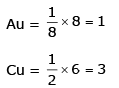Formula = AuCu3

Solid State MCQ - 1 (Advanced) - Question 22

A cubic solid is made by atoms A forming close pack arrangement, B occupying one. Fourth of tetrahedral void and C occupying half of the octahedral voids. What is the formula of compound.

Detailed Solution for Solid State MCQ - 1 (Advanced) - Question 22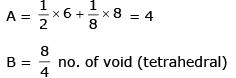= 2
C = Total no. of octahedral voids / 2 no. of octahedral voids (effective) = 1 + 12 × 1/4 = 4
Bodycenter   edgecentre C = 4/2 = 2
formula = A4B2C2

Solid State MCQ - 1 (Advanced) - Question 23

What is the percent by mass of titanium in rutile, a mineral that contain Titanium and oxygen, if structure can be described as a closet packed array of oxide ions, with titanium in one half of the octahedral holes. What is the oxidation number of titanium ?

Detailed Solution for Solid State MCQ - 1 (Advanced) - Question 23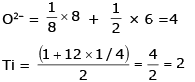TiO2 formula
Ti (At mass) = 47.88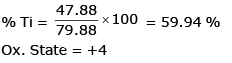Solid State MCQ - 1 (Advanced) - Question 24

Spinel is a important class of oxides consisting of two types of metal ions with the oxide ions arranged in CCP pattern. The normal spinel has one-eight of the tetrahedral holes occupied by one type of metal ion and one half of the octahedral hole occupied by another type of metal ion. Such a spinel is formed by Zn2+, Al3+ and O2-, with Zn2+ in the tetrahedral holes. Give the formulae of spinel.

Edge length, density and number of atoms per unit cell

Detailed Solution for Solid State MCQ - 1 (Advanced) - Question 24

Zn2+ = 8/8 = 1
Al3+ = 4/2 = 2
O2– = CCP (4)
ZnAl2O4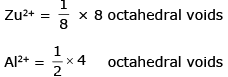O2– = CCP arrangment (1+3) = 4

Solid State MCQ - 1 (Advanced) - Question 25

Iron occurs as bcc as well as fcc unit cell. If the effective radius of an atom of iron is 124 pm. Compute the density of iron in both these structures.

Detailed Solution for Solid State MCQ - 1 (Advanced) - Question 25

In bcc z = 2